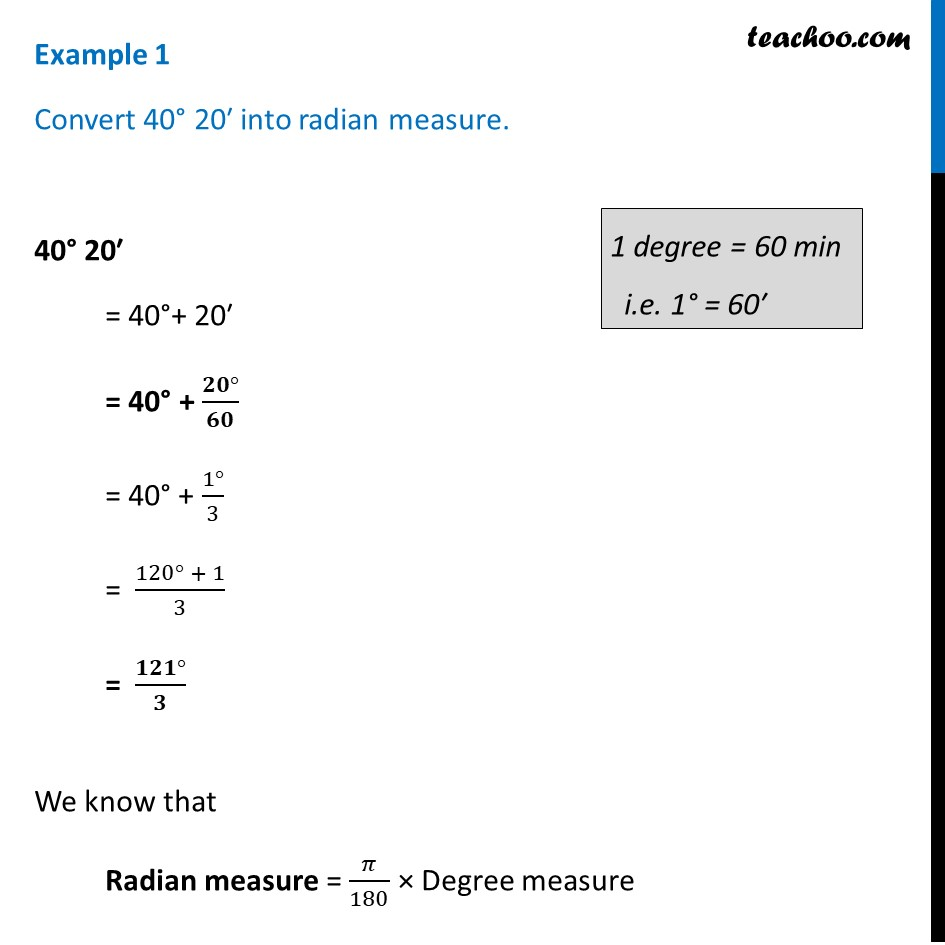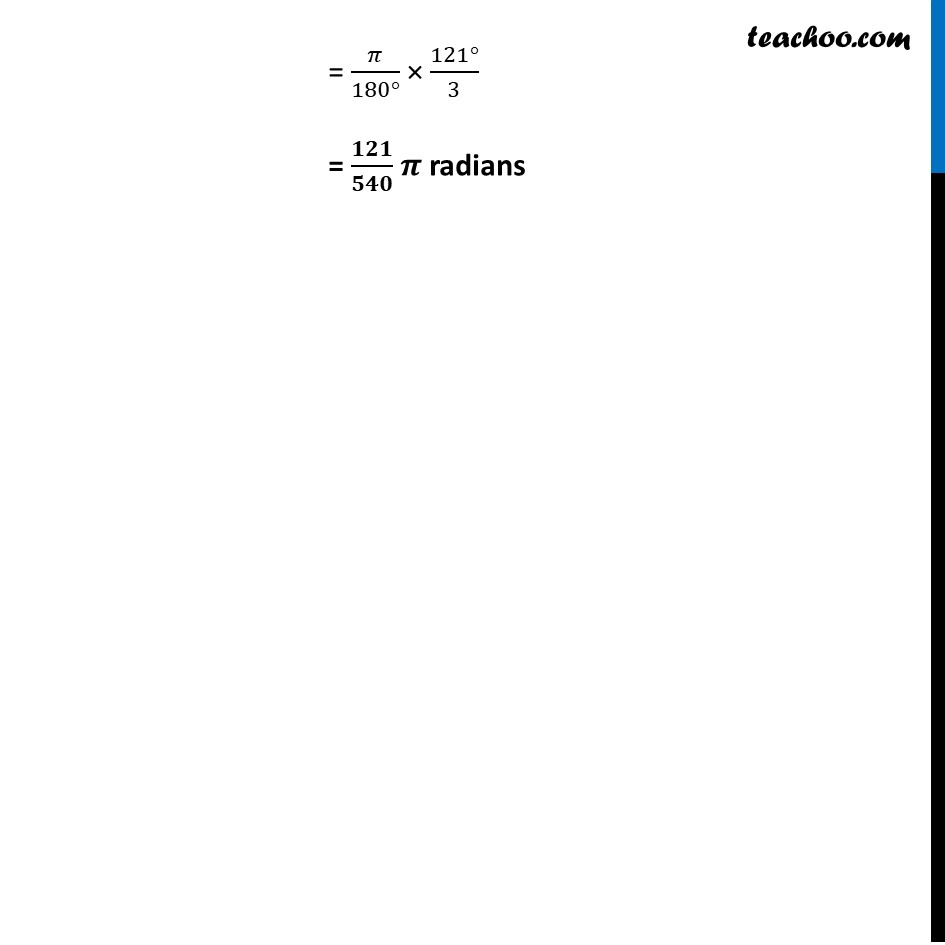Examples

Chapter 3 Class 11 Trigonometric Functions
Serial order wiseLearn in your speed, with individual attention - Teachoo Maths 1-on-1 Class

### Transcript

Example 1 Convert 40° 20′ into radian measure. 40° 20′ = 40°+ 20′ = 40° + (𝟐𝟎°)/𝟔𝟎 = 40° + (1°)/3 = (120° + 1)/3 = (𝟏𝟐𝟏°)/𝟑 We know that Radian measure = 𝜋/180 × Degree measure = 𝜋/(180°) × (121°)/3 = 𝟏𝟐𝟏/𝟓𝟒𝟎 𝝅 radians